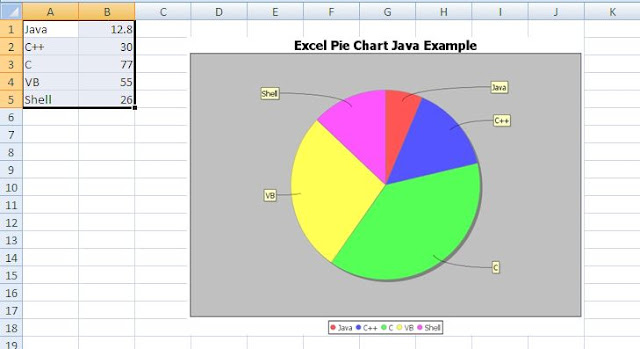# Create Pie Chart in Excel Using Java - POI JFreeChart - Example Program

## Create Pie Chart in XLSX Using POI / JFreeChart - Introduction

In the last tutorial series, we saw how to use JFreeChart with Apache POI to generate a Pie Chart in XLS file, using Java. We provided only an XLS example in the last part and in this post, we will provide XLSX example. We have a sample XLSX document with Pie Chart data source. We will read the data from the data source, create the Pie Chart and add it back to the worksheet with POI. We will provide a fully commented version of the Java Program so that you can understand what each step does. Let us get started now.

## XLSX - Create Pie Chart - Apache POI - JFreeChart - Java Example

The complete Java program that creates a Pie Chart using Apache POI / JFreeChart and writes it back to the XLSX worksheet is provided below:
```import java.io.*;
import org.apache.poi.ss.usermodel.*;
import org.apache.poi.xssf.usermodel.*;
import org.apache.poi.util.IOUtils;
import org.jfree.data.general.DefaultPieDataset;
import org.jfree.chart.ChartFactory;
import org.jfree.chart.JFreeChart;
import org.jfree.chart.ChartUtilities;
import java.util.Iterator;
public class CreatePieChartExampleXLSX {
public static void main(String[] args) throws Exception{
/* Read Excel and the Chart Data */
FileInputStream chart_file_input = new FileInputStream(new File("xlsx_pie_chart.xlsx"));
/* Read chart data from XLSX Workbook */
XSSFWorkbook my_workbook = new XSSFWorkbook(chart_file_input);
/* Read worksheet that has pie chart input data information */
XSSFSheet my_sheet = my_workbook.getSheetAt(0);
/* Create JFreeChart object that will hold the Pie Chart Data */
DefaultPieDataset my_pie_chart_data = new DefaultPieDataset();
/* We have to get the input data into DefaultPieDataset object */
/* So, we iterate over the rows and cells */
/* Create an Iterator object */
Iterator<Row> rowIterator = my_sheet.iterator();
/* Loop through worksheet data and populate Pie Chart Dataset */
String chart_label="a";
Number chart_data=0;
while(rowIterator.hasNext()) {
Row row = rowIterator.next();
//Read cells in Rows and get chart data
Iterator<Cell> cellIterator = row.cellIterator();
while(cellIterator.hasNext()) {
Cell cell = cellIterator.next();
switch(cell.getCellType()) {
case Cell.CELL_TYPE_NUMERIC:
chart_data=cell.getNumericCellValue();
break;
case Cell.CELL_TYPE_STRING:
chart_label=cell.getStringCellValue();
break;
}
}
/* Add data to the data set */
my_pie_chart_data.setValue(chart_label,chart_data);
}
/* Create a logical chart object with the chart data collected */
JFreeChart myPieChart=ChartFactory.createPieChart("Excel Pie Chart Java Example",my_pie_chart_data,true,true,false);
/* Specify the height and width of the Pie Chart */
int width=640; /* Width of the chart */
int height=480; /* Height of the chart */
float quality=1; /* Quality factor */
/* We don't want to create an intermediate file. So, we create a byte array output stream
and byte array input stream
And we pass the chart data directly to input stream through this */
/* Write chart as JPG to Output Stream */
ByteArrayOutputStream chart_out = new ByteArrayOutputStream();
ChartUtilities.writeChartAsJPEG(chart_out,quality,myPieChart,width,height);
/* We now read from the output stream and frame the input chart data */
/* We don't need InputStream, as it is required only to convert the output chart to byte array */
/* We can directly use toByteArray() method to get the data in bytes */
/* Add picture to workbook */
/* Close the output stream */
chart_out.close();
/* Create the drawing container */
XSSFDrawing drawing = my_sheet.createDrawingPatriarch();
/* Create an anchor point */
ClientAnchor my_anchor = new XSSFClientAnchor();
/* Define top left corner, and we can resize picture suitable from there */
my_anchor.setCol1(4);
my_anchor.setRow1(5);
/* Invoke createPicture and pass the anchor point and ID */
XSSFPicture  my_picture = drawing.createPicture(my_anchor, my_picture_id);
/* Call resize method, which resizes the image */
my_picture.resize();
/* Close the FileInputStream */
chart_file_input.close();
/* Write Pie Chart back to the XLSX file */
FileOutputStream out = new FileOutputStream(new File("xlsx_pie_chart.xlsx"));
my_workbook.write(out);
out.close();
}
}

```

## Datasource Input- Pie Chart XLSX Output - Example

Sample input for Pie Chart and the Output obtained using POI / JFreeChart is provided below:Create Pie Chart in XLSX Workbook - POI JFreeChart Example Output - Input / Chart Generated

The XLSX example to create Pie Chart is straight forward and can be derived easily from the XLS example for Adding Pie Chart. You may want to refer to that tutorial to get a base understanding of how we used JFreeChart and POI together to create the chart. If you have any questions, you can post it in the comments section of this post.

1.thanks buddy

2.3.IN my excel i am trying to ad multiple charts using for loop. But somehow the first chart is getting deleted.

4.Thank you so much